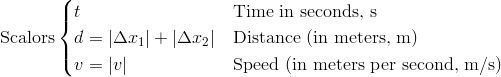Position, Distance, and DisplacementPosition and Displacement

Students will learn the meaning of an object's position, the difference between distance and displacement and some basic graphing of position vs. time.

### Key EquationsWhen beginning a one dimensional problem, define a positive direction. The other direction is then taken to be negative. Traditionally, "positive" is taken to mean "to the right"; however, any definition of direction used consistently throughout the problem will yield the right answer.

Position

Displacement

Distance

Guidance
Position is the location of the object (whether it's a person, a ball or a particle) at a given moment in time. Displacement is the difference in the object's position from one time to another. Distance is the total amount the object has traveled in a certain period of time. Displacement is a vector quantity (direction matters), where as distance is a scalar (only the amount matters). Distance and displacement are the same in the case where the object travels in a straight line and always moving in the same direction.

Example 1

Problem: An indecisive car goes 120 m North, then 30 m south then 60m North. What is the car's distance and displacement?

Solution:

Distance is the total amount traveled. Thus distance = 120 + 30 + 60 m = 210 m

Displacement is the amount displaced from the starting position. Thus displacement = 120 - 30 + 60 m = 150 m.

#### Example 2

Problem: Angrader is timed to run 24 feet in 12 seconds, what is her speed in meters per second?

Solution: